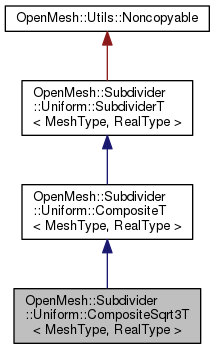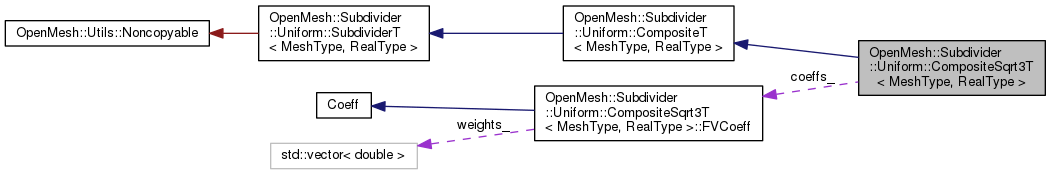OpenMesh
OpenMesh::Subdivider::Uniform::CompositeSqrt3T< MeshType, RealType > Class Template Reference

Uniform composite sqrt(3) subdivision algorithm. More...

`#include <OpenMesh/Tools/Subdivider/Uniform/CompositeSqrt3T.hh>`

Inheritance diagram for OpenMesh::Subdivider::Uniform::CompositeSqrt3T< MeshType, RealType >:[legend]
Collaboration diagram for OpenMesh::Subdivider::Uniform::CompositeSqrt3T< MeshType, RealType >:[legend]

## Classes

struct  FVCoeff
Helper class. More...

## Public Types

typedef CompositeT< MeshType, RealType > InheritedPublic Types inherited from OpenMesh::Subdivider::Uniform::CompositeT< MeshType, RealType >
typedef RealType real_t

typedef MeshType mesh_t

typedef SubdividerT< mesh_t, real_t > parent_tPublic Types inherited from OpenMesh::Subdivider::Uniform::SubdividerT< MeshType, RealType >
typedef MeshType mesh_t

typedef RealType real_t

## Public Member Functions

CompositeSqrt3T (MeshType &_mesh)

const char * name () const
Return name of subdivision algorithm.Public Member Functions inherited from OpenMesh::Subdivider::Uniform::CompositeT< MeshType, RealType >
CompositeT (MeshType &_mesh)Public Member Functions inherited from OpenMesh::Subdivider::Uniform::SubdividerT< MeshType, RealType >
virtual ~SubdividerT ()
Descructor (calls detach())

SubdividerT (void)
Constructor to be used with interface 2. More...

SubdividerT (MeshType &_m)
Constructor to be used with interface 1 (calls attach()) More...

bool operator() (MeshType &_m, size_t _n, const bool _update_points=true)
Subdivide the mesh `_m` `_n` times. More...

bool attach (MeshType &_m)
Attach mesh `_m` to self. More...

bool operator() (size_t _n, const bool _update_points=true)
Subdivide the attached `_n` times. More...

void detach (void)
Detach an eventually attached mesh. More...

## Protected Types

typedef Inherited::Coeff CoeffProtected Types inherited from OpenMesh::Subdivider::Uniform::CompositeT< MeshType, RealType >
typedef MeshType::Scalar scalar_t

typedef MeshType::VertexHandle VertexHandle

typedef MeshType::FaceHandle FaceHandle

typedef MeshType::EdgeHandle EdgeHandle

typedef MeshType::HalfedgeHandle HalfedgeHandle

## Protected Member Functions

void apply_rules (void)
Assemble here the rule sequence, by calling the constructor of the wanted rules. More...Protected Member Functions inherited from OpenMesh::Subdivider::Uniform::CompositeT< MeshType, RealType >
bool prepare (MeshType &_m)

bool subdivide (MeshType &_m, size_t _n, const bool _update_points=true)
Subdivide mesh `_m` `_n` times.

bool cleanup (MeshType &_m)
Cleanup mesh after usage, e.g. remove added properties.

void commit (MeshType &_m)
Move vertices to new positions after the rules have been applied to the mesh (called by subdivide()). More...

void corner_cutting (HalfedgeHandle _heh)
Corner Cutting.

VertexHandle split_edge (HalfedgeHandle _heh)
Split Edge.

void Tvv3 ()
Split Face, using Vertex information (1-3 split)

void Tvv4 ()
Split Face, using Vertex information (1-4 split)

void Tfv ()
Split Face, using Face Information.

void FF ()
Face to face averaging.

void FFc (Coeff &_coeff)
Weighted face to face averaging.

void FFc (scalar_t _c)
Weighted face to face averaging.

void FV ()
Face to vertex averaging.

void FVc (Coeff &_coeff)
Weighted face to vertex Averaging with flaps.

void FVc (scalar_t _c)
Weighted face to vertex Averaging with flaps.

void FE ()
Face to edge averaging.

void VF ()
Vertex to Face Averaging.

void VFa (Coeff &_coeff)
Vertex to Face Averaging, weighted.

void VFa (scalar_t _alpha)
Vertex to Face Averaging, weighted.

void VV ()
Vertex to vertex averaging.

void VVc (Coeff &_coeff)
Vertex to vertex averaging, weighted.

void VVc (scalar_t _c)
Vertex to vertex averaging, weighted.

void VE ()
VE Step (Vertex to Edge Averaging)

void VdE ()
Vertex to edge averaging, using diamond of edges.

void VdEc (scalar_t _c)
Weighted vertex to edge averaging, using diamond of edges.

void VdEg (Coeff &_coeff)
Weigthed vertex to edge averaging, using diamond of edges for irregular vertices. More...

void VdEg (scalar_t _gamma)
Weigthed vertex to edge averaging, using diamond of edges for irregular vertices. More...

void EF ()
Edge to face averaging.

void EV ()
Edge to vertex averaging.

void EVc (Coeff &_coeff)
Weighted edge to vertex averaging.

void EVc (scalar_t _c)
Weighted edge to vertex averaging.

void EdE ()
Edge to edge averaging w/ flap rule.

void EdEc (scalar_t _c)
Weighted edge to edge averaging w/ flap rule.

## Protected Attributes

OpenMesh::Subdivider::Uniform::CompositeSqrt3T::FVCoeff coeffs_

## Detailed Description

### template<typename MeshType, typename RealType = float> class OpenMesh::Subdivider::Uniform::CompositeSqrt3T< MeshType, RealType >

Uniform composite sqrt(3) subdivision algorithm.

## Member Function Documentation

template<typename MeshType , typename RealType = float>
 void OpenMesh::Subdivider::Uniform::CompositeSqrt3T< MeshType, RealType >::apply_rules ( void )
inlineprotectedvirtual

Assemble here the rule sequence, by calling the constructor of the wanted rules.

The documentation for this class was generated from the following file:

Project OpenMesh, ©  Computer Graphics Group, RWTH Aachen. Documentation generated using doxygen .